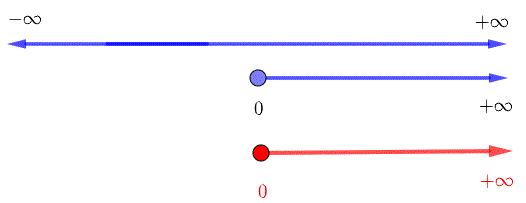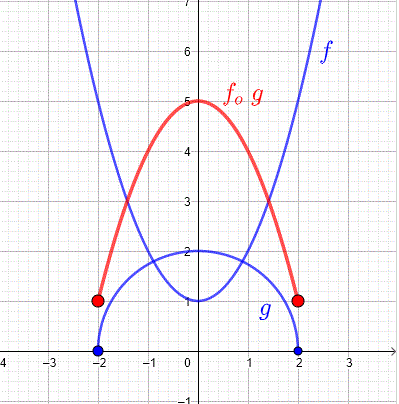# Composition of Functions Examples

The definition and the properties of the composition of functions are discussed through examples with detailed solutions and explanations.

## Definition of Composition of Functions

Let $$f$$ and $$g$$ be two functions. If we let function $$f$$ take as input function $$g$$ , see diagram below, the resulting function is called the composite function or composition of $$f$$ and $$g$$ denoted by $$f_o g$$ and is defined by

$(f_o g)(x) = f(g(x))$

This composite function is defined if $$x$$ is in the domain of $$g$$ and $$g(x)$$ is in the domain of $$f$$. (see digram below).## Examples with Solutions

### Example 1: Composition of Functions Given Tables of Values

Functions $$f$$ and $$g$$ are defined by their tables as follows
 $$x$$ $$f(x)$$ $$x$$ $$g(x)$$ 3 4 2 6 5 7 6 3 6 8 7 9 8 11 8 5

1) Find the values of:
a) $$(f_o g)( 2 )$$ ,      b) $$(f_o g)( 6 )$$ ,      c) $$(f_o g)( 7 )$$ ,      d) $$(f_o g)( 8 )$$
e) $$(g_o f)( 3 )$$ ,      f) $$(g_o f)( 5 )$$ ,      g) $$(g_o f)( 6 )$$ ,      h) $$(g_o f)( 8 )$$
i) $$(g_o g)( 2 )$$
2) Find the domain of $$f_o g$$ and $$g_o f$$

### Solution to Example 1

1)
a) Use definition of composition of functions to write
$$(f_o g)( 2 ) = f(g(2))$$
Use table to find the value of $$g(2) = 6$$
Substitute back in $$f(g(2))$$ to write
$$(f_o g)( 2 ) = f(g(2)) = f(6)$$
Use table to evaluate $$f(6)$$
$$(f_o g)( 2 ) = f(g(2)) = f(6) = 8$$
Use similar steps as above
b) $$(f_o g)( 6 ) = f(g(6)) = f(3) = 4$$
c) $$(f_o g)( 7 ) = f(g(7)) = f(9) =$$ undefined
d) $$(f_o g)( 8 ) = f(g(8)) = f(5) = 7$$
e) $$(g_o f)( 3 ) = g(f(3)) = g(4) =$$ undefined
f) $$(g_o f)( 5 ) = g(f(5)) = g(7) = 9$$
g) $$(g_o f)( 6 ) = g(f(6)) = g(8) = 5$$
h) $$(g_o f)( 8 ) = g(f(8)) = g(11) =$$ undefined
i) $$(g_o g)( 2 ) = g(g(2)) = g(6) = 3$$
2)
Use the results in part 1) to write the ordered pairs defining $$g_o f$$ and $$f_o g$$ and then deduce the domain.
$$g_o f : \{(2,6),(6,4),(8,7)\}$$ , hence the domain of $$g_o f$$ is given by: {2,6,8}
$$f_o g : \{(5,9),(6,5)\}$$ , hence the domain of $$f_o g$$ is given by: {5,6}

### Example 2: Composition of Functions Given by their Graphs

Functions $$f$$ and $$g$$ are given by their graphs shown belowFind the values of:
a) $$(f_o g)( -2 )$$      b) $$(f_o g)( 0 )$$      c) $$(f_o g)( 4 )$$      d) $$(f_o g)( 8 )$$      e) $$(g_o f)( 2 )$$      f) $$(g_o f)( 0 )$$

### Solution to Example 2

a) Use definition of composition of functions to write
$$(f_o g)( -2 ) = f(g(-2))$$
Use the graph of g to find the value of g(-2) = - 3
Substitute back in $$f(g(-2))$$ to write
$$(f_o g)( -2 ) = f(g(-2)) = f(-3)$$
Use the graph of f to evaluate $$f(-3)$$
$$(f_o g)( -2 ) = f(g(-2)) = f(-3) = 9$$
Use similar steps as above to evaluate the following
b) $$(f_o g)( 0 ) = f(g(0)) = f(-2) = 4$$
c) $$(f_o g)( 4 ) = f(g(4)) = f(0) = 0$$
d) $$(f_o g)( 8 ) = f(g(8)) = f(2) = 4$$
e) $$(g_o f)( 2 ) = g(f(2)) = g(4) = 0$$
f) $$(g_o f)( 0 ) = g(f(0)) = g(0) = -2$$

### Example 3: Composition of Functions Given by their Formulas

Functions $$f$$ and $$g$$ are defined by the formulas: $$f(x) = 2x + 1$$ and $$g(x) = - x + 1$$
a) Find the composite function $$(g_o f)( x )$$.
b) Find the composite function $$(f_o g)( x )$$.

### Solution to Example 3

a)
Use the definition of composition of functions to write
$$(g_o f)( x ) = g(f(x))$$
Express $$g(f(x))$$ in terms of $$f(x)$$
$$(g_o f)( x ) = g(f(x)) = - ( f(x) ) + 1$$
Substitute $$f(x)$$ by its formula
$$(g_o f)( x ) = g(f(x)) = - ( 2x + 1 ) + 1$$
Simplify
$$(g_o f)( x ) = g(f(x)) = - ( 2x + 1 ) + 1 = - 2 x$$
b)
Use definition of composition of functions to write
$$(f_o g)( x ) = f(g(x))$$
Express $$f(g(x))$$ in terms of $$g(x)$$
$$(f_o g)( x ) = f(g(x)) = 2 g(x) + 1$$
Substitute $$g(x)$$ by its formula and simplify
$$(f_o g)( x ) = f(g(x)) = 2 g(x) + 1 = 2(- x + 1) + 1 = -2x + 3$$
Note that $$(g_o f)( x ) \ne (f_o g)( x )$$ , which means that the composition of functions is not commutative.

### Example 4: Composition of Functions and their Domains

Functions $$f$$ and $$g$$ are defined by the formulas: $$f(x) = 2x + 1$$ and $$g(x) = \sqrt{x - 1}$$
Find the composite function $$(g_o f)( x )$$ and and its domain.

### Solution to Example 4

$$(g_o f)( x ) = g(f(x)) = \sqrt{f(x) - 1} = \sqrt{2x+1 - 1} =\sqrt{2x}$$
Two conditions for the domain of $$(g_o f)$$
1) $$x$$ must be in the domain of $$f$$ which is given by the interval : $$(-\infty , +\infty)$$
2) $$f(x)$$ must be in the domain of $$g$$ which is the domain of $$g(f(x))$$ .
The domain of $$g(f(x)) = \sqrt{2x}$$ is found by solving the inequality: $$2x \ge 0$$ wihich has the solution set given by the interval: $$[0 , + \infty)$$
The domain of $$(g_o f)$$ is given by the intersection (red) of the sets in 1) and 2) ( in blue) :
$$[0 , + \infty)$$### Example 5: Composition of Functions and their Domains

Functions $$f$$ and $$g$$ are defined by the formulas: $$f(x) = x^2 + 1$$ and $$g(x) = \sqrt{4-x^2}$$
1) Find the composite function $$(f_o g)( x )$$ and and its domain.
2) Graph function $$f$$, $$g$$ and $$f_o g$$ in the same system of coordinates.

### Solution to Example 5

1)
$$(f_o g)( x ) = f(g(x)) = (g(x))^2 + 1 = (\sqrt{4-x^2})^2 + 1 = 5 - x^2$$
Two conditions for the domain of $$(f_o g)$$
1) $$x$$ must be in the domain of g which is found by solving the inequality $$4-x^2 \ge 0$$. The domain of g is given by the interval : $$[-2, + 2]$$
2) $$g(x)$$ must be in the domain of f which is the domain of $$f(g(x))$$ which is the interval $$(-\infty , +\infty)$$.
The domain of $$(f_o g)$$ is given by the intersection (red) of the sets in 1) and 2) (blue) :
$$[-2 , + 2]$$2)
Below are shown the graphs of $$f$$, $$g$$ and $$f_o g$$.## Properties of Composite Functions

### Property 1

In general $$(f_o g)(x) \ne (g_o f)(x)$$ and therefore the composition of functions is
not commutative.
Example 3 above already shows that the composition of functions is not commutative.

### Example 6: The composition is not commutative

Let $$f(x) = x^2 - 1$$ and $$g(x) = 2x$$
Show that $$(f_o g)(x) \ne (g_o f)(x)$$

### Solution to Example 6

$$(f_o g)(x) = f(g(x)) = (g(x))^2 - 1 = (2x)^2 - 1 = 4x^2 - 1$$
$$(g_o f)(x) = g(f(x)) = 2 f(x) = 2 (x^2 - 1) = 2x^2 - 2$$
Therefore $$(f_o g)(x) \ne (g_o f)(x)$$ and the composition of functions is not commutative.

### Property 2

Let $$f, g$$ and $$h$$ be three functions, $$f_o (g_o h) = (f_o g)_o h$$ and therefore the composition of funtions is
associative.

### Example 7: The composition of Functions is associative

Show that $$(f_o (g_o h))(x) = ((f_o g)_o h)(x)$$

### Solution to Example 7

1) Left side
Use definition of composition to write
$$(f_o (g_o h))(x) = f((g_o h)(x))$$
Use definition of composition again to write
$$= f(g(h(x))$$
2) Right side
Use definition of composition to write
$$((f_o g)_o h)(x) = (f_o g)(h(x))$$
Use definition of composition to write
$$= f(g(h(x)))$$
Therefore
$$(f_o (g_o h))(x) = ((f_o g)_o h)(x)$$

### Property 3

If $$f$$ and $$g$$ are
invertible, then $$(f_o g)^{-1} = g^{-1}_o f^{-1}$$

### Example 8: The inverse of the composition of Functions

Let $$f(x) = \dfrac{1}{x-1}$$ and $$g(x) = - x + 5$$
Show that $$(f_o g)^{-1} = g^{-1}_o f^{-1}$$

### Solution to Example 8

We first calculate $$(f_o g)(x)$$ and then its inverse $$(f_o g)^{-1}(x)$$
$$(f_o g)(x) = f(g(x)) = \dfrac{1}{g(x)-1} = \dfrac{1}{-x + 5 -1} = \dfrac{1}{-x + 4}$$
$$(f_o g)^{-1}(x) = - \dfrac{1}{x} + 4$$
We now calculate the inverses $$f^{-1}(x)$$ , $$g^{-1}(x)$$ and then calculate the composition $$(g^{-1}_o f^{-1})(x)$$.
$$f^{-1}(x) = \dfrac{1}{x} + 1$$
$$g^{-1}(x) = - x + 5$$
$$(g^{-1}_o f^{-1})(x) = g^{-1}(f^{-1}(x)) = - (f^{-1}(x)) + 5 = - ( \dfrac{1}{x} + 1) + 5 = - \dfrac{1}{x} + 4$$
We conclude that
$$(g^{-1}_o f^{-1})(x) = (f_o g)^{-1}(x)$$